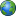Home | News Update | English Language | Mathematics | Physics | Biology | Geography | Forum | About Us
Fast & Easy Contact??08080092411 ||Coming soon..
Mathematics_pictures
think
lenght
learn
Maths Literacy - Ratios and Percentages
Sequences and series
estimating surds
factorisation
logarithms
rounding off
word problems
standard formIdentity 1

a/b×c/d = ac/bd (b≠0;d≠0)

a/b+c/b = a+c/b (b≠0)

a/b÷c/d = a/b×d/c = ad/bc (b≠0;c≠0;d≠0)

Note: dividing by a fraction is the same as multiplying by the reciprocal of the fraction. In some cases of simplifying an algebraic expression, the expression will be a fraction. For example, x2+3x/x+3

has a quadratic binomial in the numerator and a linear binomial in the denominator. We have to apply the different factorisation methods in order to factorise the numerator and the denominator before we can simplify the expression. x2+3x/x+3 = x(x+3)x+3 = x(x≠−3)

If x = −3 then the denominator, x+3=0, and the fraction is undefined.

Question Simplify: ax−b+x−ab/ax2−abx, (x≠0;x≠b).
Use grouping to factorise the numerator and take out the common factor ax in the denominator

(ax−ab)+(x−b)/ax2−abx = a(x−b)+(x−b)/ax(x −b)

Take out common factor (x−b) in the numerator =(x−b)(a+1)/ax(x−b)

Cancel the common factor in the numerator and the denominator to give the final answer =a+1/ax

Exercise 1 – Simplifying Fractions

Problem 1:

Simplify (assume all denominators are non- zero):

1. 3a/15

2. 2a+10/4

3. 5a+20/a+4Share Your Story With The World Part of Uzomedia site) site owned and manage by Uzomedia..pacman, rainbows, and roller s